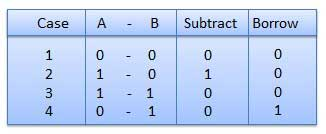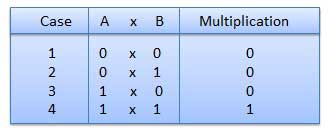# Binary Number System

Digital computer represents all kinds of data and information in the binary system. Binary Number System consists of two digits 0 and 1. Its base is 2. Each digit or bit in binary number system can be 0 or 1. A combination of binary numbers may be used to represent different quantities like 1001. The positional value of each digit in binary number is twice the place value or face value of the digit of its right side. The weight of each position is a power of 2.example for binary addittion:### Binary subtraction:example for binary subtraction:### Binary multiplication:example for binary multiplication:### Binary division:\

example for binary division:### Binary Number conversions:binary to decimal conversionbinary to hexadecimal conversionbinary to octal conversion

Some important number systems are as follows.

To gain more knowledge about hardware and software just click here

You can share your feedback (both positive and negative) by mailing to [email protected]. It will help us to improve our site.We will respond your mail within 24 hours.